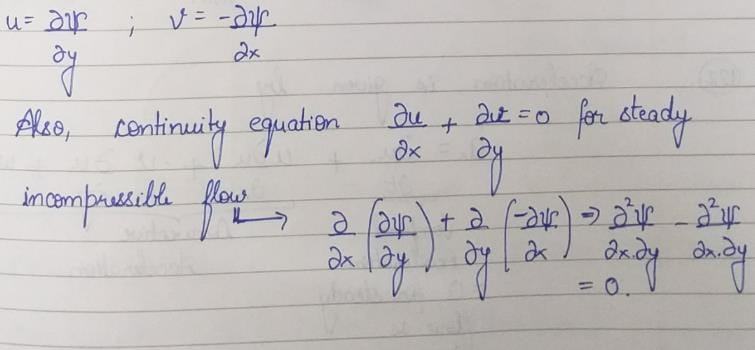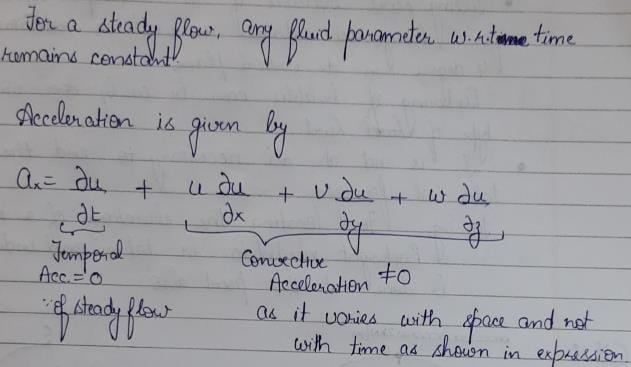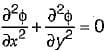# Test: Fluid Flow Kinematics - 2

## 10 Questions MCQ Test Topicwise Question Bank for Civil Engineering | Test: Fluid Flow Kinematics - 2

Description
Attempt Test: Fluid Flow Kinematics - 2 | 10 questions in 30 minutes | Mock test for Civil Engineering (CE) preparation | Free important questions MCQ to study Topicwise Question Bank for Civil Engineering for Civil Engineering (CE) Exam | Download free PDF with solutions
QUESTION: 1

Solution:
QUESTION: 2

Solution:
QUESTION: 3

### A stream function is

Solution:

A stream function is defined by following characteristic:
The partial derivative of stream function w.r.t y will give velocity in x-direction.
The partial derivative of stream function w.r.t x will give velocity in negative y-direction.
It is valid for steady, incompressible flow since, is satisfies the continuity equationQUESTION: 4

The continuity equation for steady incompressible flow is expressed in vector notation as

Solution:
QUESTION: 5

In a converging steady flow there is

Solution:QUESTION: 6

In two dimensional flow, the equation of a streamline is given as

Solution:
QUESTION: 7

The concept of stream function which is based on the principle of continuity is applicable to

Solution:

Velocity potential function is valid for 3-dimensional flow while stream function is valid for 2 dimensional flow.

QUESTION: 8

Which of the following velocity potentials satisfies continuity equation?

Solution:

For the velocity potential function to satisfy continuity equation:Where φ is velocity potential, φ = x2 - y2 satisfies this equation

QUESTION: 9

In a two dimensional incompressible steady flow around an airfoil, the stream lines are 2 cm apart at a great distance from the airfoil, where the velocity is 30 m/sec. The velocity near the airfoil, where the stream lines are 1.5 cm apart, is

Solution:

V1y1 = V2y2
⇒ 30 x 2 = V2 x 1.5 ⇒ v2 = 40 cm/s

QUESTION: 10

The velocity potential function for a source varies with distance r as

Solution:

Velocity at any point r in the flow field of source is given by, Vr = q/2πr.Use Code STAYHOME200 and get INR 200 additional OFF Use Coupon Code